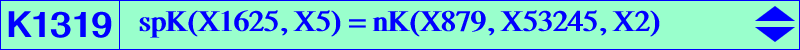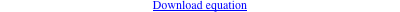too complicated to be written here. Click on the link to download a text file.X(2), X(3), X(4), X(879), X(14355), X(14592), X(15412), X(43665), X(53173), X(53174), X(53175) infinite points of pK(X6, X1625) other points below Geometric properties :K1319 is a MacBeath cubic as in Table 80. It is a non-isogonal nK with pole X(879) and root the barycentric quotient R = X(5) ÷ X(511) = X(53245), on the lines {X6,X264}, {X22,X98}, {X51,X324}, etc, SEARCH = -1.24130261280343. K1319 contains the reflection P2 of X(1625) in X(5). P2 lies on the lines {X2,X98},{X4,X290},{X5,X217},{X51,X324},{X68,X248}, etc, SEARCH = 1.04598827213825. P2 is the barycentric quotient X(879) ÷ X(15412) = X15412*. Hence, K1319 meets (O) at the same points as pK(X6, P2). K1319 contains P1 = X(879) ÷ X(4) = X4*, on the lines {X2, X647}, {X3, X525}, {X97, X2525}, {X98, X1297}, etc, SEARCH = -118.722232092805. P1 and P2 are now X(53173) and X(53174) in ETC. Other points on K1319 : P3 = {4, 14592} ∩ {14355, P1}, SEARCH = 6.73132265277523 P4 = {3, 14355} ∩ {14592, 43665}, SEARCH = 0.0358702537707717 P5 = {3, 14592} ∩ {14355, 43665} = P4*, SEARCH = 4.59250720134740 P6 = {4, 14355} ∩ {14592, P1} = P3*, SEARCH = 0.0793238046066764